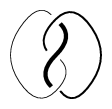# Fox-n-colouring

A colouring of a non-oriented link diagram (cf. also Knot and link diagrams), leading to an Abelian group invariant of links in $\mathbf{R}^3$ (cf. also Link). It was introduced by R.H. Fox around 1956 to visualize dihedral representations of the knot group [a1] (cf. also Knot and link groups). Using $3$-colourings is, probably, the simplest method of showing that the trefoil knot is non-trivial (see Fig.a1).

One says that a link (or tangle) diagram, $D$, is $n$-coloured if every arc is coloured by one of the numbers $0,\ldots,(n-1)$ in such a way that at each crossing the sum of the colours of the undercrossings is equal to twice the colour of the overcrossing modulo $n$. The set of $n$-colourings forms an Abelian group, denoted by $\text{Col}_n(D)$. This group can be interpreted using the first homology group (modulo $n$) of the double branched cover of $S^3$ with the link as the branched point set. The group of $3$-colourings is determined by the Jones polynomial (at $t=e^{2\pi i/6}$), and the group of $5$-colourings by the Kauffman polynomial (at $a=1$, $z = 2\cos(2\pi/5)$), [a2]. The $n$-moves preserve the group of $n$-colourings and $3$-moves lead to the Montesinos–Nakanishi conjecture (cf. Tangle move).Figure: f130220a

The linear space of $p$-colourings of the boundary points of an $n$-tangle has a symplectic form (cf. also Symplectic structure), so that tangles correspond to Lagrangian subspaces (i.e. maximal totally degenerate subspaces) of the symplectic form.

The Alexander module is a generalization of the group of $n$-colourings.

How to Cite This Entry:
Fox-n-colouring. Encyclopedia of Mathematics. URL: http://encyclopediaofmath.org/index.php?title=Fox-n-colouring&oldid=40115
This article was adapted from an original article by Jozef Przytycki (originator), which appeared in Encyclopedia of Mathematics - ISBN 1402006098. See original article